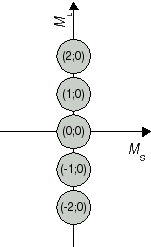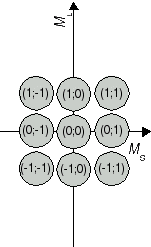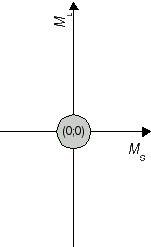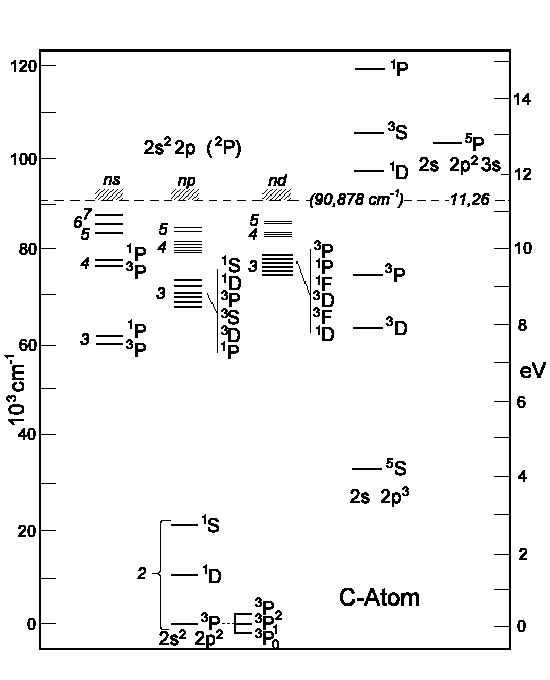From Carbon To NeonCarbon:
z = 6

 n s p L 2 Ýß Ý Ý K 1 Ýß C

1s2 2s2 2p2

The total spin can be 0 or 1. The total orbital momentum can be 0, 1 or 2 since either electron possesses an orbital momentum of 1. |l1l2| ≤ L ≤ l1 + l2. Now let's try to explain possible configurations with l ≠ 0. Primarily, both p-electrons have common quantum numbers n and l; therefore only the quantum numbers (ml1, ms1) and (ml2, ms2) remain to be considered. ml1 and ml2 may be equal to -1, 0, +1 and ms1 and ms2 may be equal to -½, +½.

Table 1 contains the results for the total orbit momentum ML and the total spin MS. These values represent the sum of the orbit momenta ml and of the spins ms of the two electrons.

• Six combinations are excluded due to Pauli's principle which forbids that one pair of electrons is described by identical sets of quantum numbers. "X" appears in the respective cells.
• As we have only one kind of electrons and our labelling "1" and "2" is fictional, there are only 15 instead of 30 possible combinations. The cell's content "s.a." (for "see above") indicates that the combination is described in a cells above the table's diagonal.

 e- 1. 2. ml -1 0 +1 ms -½ ½ -½ ½ -½ ½ -1 -½ X ( -2; 0) (-1;-1) (-1; 0) ( 0;-1) ( 0; 0) ½ s.a. X (-1; 0) (-1; 1) ( 0; 0) ( 0; 1) 0 -½ s.a. s.a. X ( 0; 0) ( 1;-1) ( 1; 0) ½ s.a. s.a. s.a. X ( 1; 0) ( 1; 1) +1 -½ s.a. s.a. s.a. s.a. X ( 2; 0) ½ s.a. s.a. s.a. s.a. s.a. X
Table 1: Determination of resultant pairs of ML and MS

The recieved combinations (ML;MS) belong to atomic states 2S+1L J. In a second step, we determine the respective states. We start with the highest value for L, i.e. ML = 2. From this ML, we conclude that the two electrons produce an atomic state with L = 2 which is denoted with a capital D in the term symbol. Both extremes, ML = 2 and ML = -2 are combined with MS = 0, i.e. an antiparallel orientation of the two electron spins. Therefore, we have state 1D. The calculated pairs (2;0), (1;0), (0;0), (-1;0) and (-2;0) are special cases for the carbon atom in state 1D (compare Fig. 1a). As next value, ML = 1 has been obtained, for example in (ML;MS) = (1;1). This combination is one out of nine cases which emerge from assuming an atomic state with L = 1 and S = 1. The respective state is denoted as 3P state. (compare Figure 1b). So far, 5 + 9 = 14 of our set of 15 have been identified as cases of two states. The remaining pair (0;0) is the only case for a state with L = 0 and S = 0 (compare Fig 1c); in other words, an atomic state 1S0.Fig. 1a J=2 S=0    1D2Fig. 1b J=1 S=1    3P2,1,0Fig. 1c J=0 S=0    1S0

Therefore, dependent on the distribution of electrons and their spins, the carbon atom exists in states denoted by the term symbols 1S0, 3P0, 3P1, 3P2 and 1D2. These symbole have been introduced in table 2.

 e- 1. 2. ml -1 0 +1 ms -½ ½ -½ ½ -½ ½ -1 -½ X 1D 3P 3P 3P 1S ½ s.a. X 1D 3P 3P 3P 0 -½ s.a. s.a. X 1D 3P 3P ½ s.a. s.a. s.a. X 1D 3P +1 -½ s.a. s.a. s.a. s.a. X 1D ½ s.a. s.a. s.a. s.a. s.a. X
Table 2: The combinations are representations of three atomic states

According to Hund's rule (1) the ground state is 3P and according to rule (2) is the 3P0 state.

 C : K 2s2 2p2  ≡3P0

Other energy terms than 1S, 3P und 1D, i.e. the excited the carbon atom, are represented on the next illustration. To the right one can see the energy levels which are obtained by (2s) electron excitation.Figure: Energy levels of Carbon atoms.  To the left: the (2p)-electron excitation. To the right: the (2s)-electron excitation.

The ground state of C-atom is the combination of two (np)-electrons. The table below lists the terms for an atom with three (np)-electrons, i.e. p3 which leads to the states 4S, 2P and 2D.

 Configuration Term Configuration Term s  s2 p, p5 p2, p4 p3 2S  1S  2P  1S, 1D, 3P  4S, 2P, 2D d, d9 d2, d8 d3, d7 d4, d6 d5 2D  1S, 1D, 1G, 3P, 3F  2D, 2F, 2G, 2H, 4P, 4F  1S, 1D, 1F,1G,1I,3P,3D,3F,3G,3H,5D  2S, 2P, 2D,2F,2G,2H,2I,4P,4D,4F,4G,6S

Nitrogen:
z = 7

 n s p L 2 Ýß Ý Ý Ý K 1 Ýß N

(1s2) ( 2s2) ( 2p3)

According to Hunds rule spins should be directed as parallel to each other as possible. So that the total spin will be S = 3/2. According to the above table 3 terms 4S, 2P, 2D is the combination of three p-electrons. And the state is:

 4S3/2

Oxygen:
z = 8

 n s p L 2 Ýß Ýß Ý Ý K 1 Ýß O

(1s2) ( 2s2) ( 2p4)

The above-mentioned considerations would be very difficult to apply to this atom having 4 p-electrons: we will consider another way of 4 p-electrons combination here:

We've known that the shell is fulfilled with 6 p-electrons (p6) and also L = 0 and S = 0. We will represent our Oxygen atom as 2 electrons of (p6)-shell would be absent. Now these missing electrons or also holes are combined into "2-holes configuration" since holes have also spin ½. Therefore we have as for Carbon atom the following possible states 1S, 3P, 1D. The highest spin (Hunds rule (1)) is S = 1 i.e. 3P is the ground state and total kinetic moment J is for the ground state (Hunds rule (2)) J = L + S = 2. And finally:

 3P2

Fluorine:
z = 9

 n s p L 2 Ýß Ýß Ýß Ý K 1 Ýß F

(1s2) ( 2s2) (2p5: 2P3/2

There is no one p-electron i.e. L = 1 and S = ½.

J = ½ and 3/2 are possible. According to Hunds rule (2) the higher J-value is possible for the ground state:

 2P3/2

Neon:
z = 10

 n s p L 2 Ýß Ýß Ýß Ýß K 1 Ýß Ne

(1s2) (2s2) (2p6: 1S0

2p-shell is full ! High ionization energy 21,6 eV.Auf diesem Webangebot gilt die Datenschutzerklärung der TU Braunschweig mit Ausnahme der Abschnitte VI, VII und VIII.